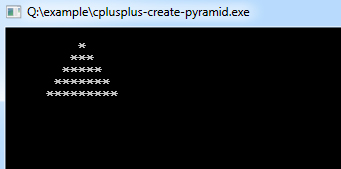## C++ Programming Examples Tutorial Index

This C++ program is used to demonstrates how to create a triangular pattern.

Example:

``````#include <iostream>
using namespace std;

int main()
{
int i,j,k,space=10; // to print the pyramid in center, you can also increase the # of spaces

for (int i=0;i<=5;i++)
{
for (int k=0;k<space;k++)
{
cout<<" ";
}
for (int j=0;j<2*i-1;j++)
{
cout<<"*";
}
space--;
cout<<endl;
}
cin.get(); /*use this to wait for a keypress*/
}
``````

Program Output:Explanation:

First of all, you have to include the iostream header file using the "include" preceding by # which tells that hat the header file needs to be process before compilation, hence named preprocessor directive. Now, for removing naming conflict you can use namespace statement within a program.

Then the main() function is declared with return type as integer. Now you have to declare four integer type variables name as 'i', 'j', 'k' and 'space' and assign the value of space as 10. Now you have to implement two for() loop, first one counts the iteration from i=0 to i<=5. The second for loop, which is a nested for() loop counts the iteration from k = 0 to k<space.

Another for loop will be required which is the used to print the asterisk using the cout<<""; statement. That for loop goes from j=0 till j<2*i-1. Then the value of space needs to be decreased using the decrement operator since the space variable is counting the number of spaces to be accommodated before and after the '*' asterisk symbol to create the pattern. Then finally the cin.get() function is used to wait for the user to press any key.